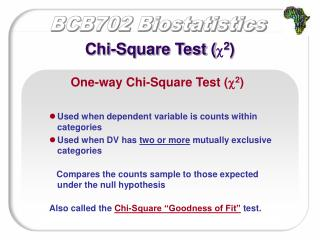DownloadDownload PresentationChi-Square Test ( c 2 )

# Chi-Square Test ( c 2 )

Download Presentation## Chi-Square Test ( c 2 )

- - - - - - - - - - - - - - - - - - - - - - - - - - - E N D - - - - - - - - - - - - - - - - - - - - - - - - - - -
##### Presentation Transcript

1. Chi-Square Test (c2) One-way Chi-Square Test (c2) • Used when dependent variable is counts within categories • Used when DV has two or more mutually exclusive categories Compares the counts sample to those expected under the null hypothesis Also called the Chi-Square “Goodness of Fit” test.

2. Chi-Square Test (c2) One-way Chi-Square Test (c2) EXAMPLE Which power would you rather have: flight, invisibility, or x-ray vision? Is this difference significant, or is just due to chance?

3. Chi-Square Test (c2) EXAMPLE One-way Chi-Square Test (c2)

4. Chi-Square Test (c2) EXAMPLE One-way Chi-Square Test (c2)

5. Chi-Square Test (c2) EXAMPLE One-way Chi-Square Test (c2)

6. Chi-Square Test (c2) EXAMPLE One-way Chi-Square Test (c2)

7. Chi-Square Test (c2) One-way Chi-Square Test (c2) Review: Steps: 1) State hypotheses 2) Write observed and expected frequencies 3) Get c2 by summing up relative squared deviations 4) Use Table I to get critical c2

8. Chi-Square Test (c2)

9. Chi-Square Test (c2) Two-factor Chi-Square Test (c2) • Used to test whether two nominal variables are independent or related • E.g. Is gender related to socio-economic class? • Compares the observed frequencies to the frequencies expected if the variables were independent • Called a chi-squared test of independence • Fundamentally testing, “do these variables interact”?

10. Chi-Square Test (c2) Two-factor Chi-Square Test (c2) A 1999 poll sampled people’s opinions concerning the use of the death penalty for murder when given the option of life in prison instead. 800 people were polled, and the number of men and women supporting each penalty were tabulated. Contingency table: shows contingency between two variables Are these two variables (gender, penalty preference) independent??

11. Chi-Square Test (c2) EXAMPLE Two-factor Chi-Square Test (c2) H0:distribution of female preferences matches distribution of male preferences HA:female proportions do not match male proportions

12. Chi-Square Test (c2) EXAMPLE Two-factor Chi-Square Test (c2) We want to test whether the distribution of preferences for men and women is the same (e.g. no interaction effects) . We need to look at the marginal totals to get our expected frequencies

13. Chi-Square Test (c2) EXAMPLE Two-factor Chi-Square Test (c2)

14. Chi-Square Test (c2) EXAMPLE Two-factor Chi-Square Test (c2)

15. Chi-Square Test (c2) Two-factor Chi-Square Test (c2) EXAMPLE

16. Steps: 1) State hypotheses 2) Get expected frequencies 3) Get c2 by summing up relative squared deviations 4) Use table to get critical c2 Chi-Square Test (c2) Two-factor Chi-Square Test (c2)

17. Suppose we want to determine if there is any relationship between level of education and medium through which one follows current events. We ask a random sample of high school graduates and a random sample of college graduates whether they keep up with the news mostly by reading the paper or by listening to the radio or by watching television. Chi-Square Test (c2) PRACTICE

18. = 17.89 df = (2)*(1) = 2 Chi-Square Test (c2) PRACTICE